# Computing Vegetation Health

### Overview

Explore using units for calculations with Normalized Difference Vegetation Index (NDVI). NDVI is a ratio of different light wavelength reflectance which can be used to map the density of green vegetation.

### Student Directions

#### Background

You can do math with units just like you do it with numbers. Fractions made of two different units with equal magnitudes can be made into fractions equal to 1. For example, 1 meter = 100 cm. Since they are equal, the following fractions are equal to 1. These are called unit multipliers.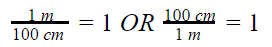When you multiply a number by 1, the answer is the original number. This works for fractions equal to 1.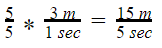Knowing this, the units can be canceled when doing calculations, just like numbers. This can be used with unit multipliers to convert units. The answer will have different units but it is still the same actual amount. For example, 3 m per second is equal to how many centimeters per second?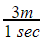needs to be converted to centimeters per second.

Use the unit multiplier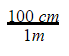and cancel units.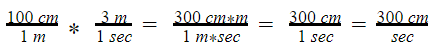This is how you determine the units for the answer to a problem. Sometimes, all the units will cancel out giving a number with no units. This often happens with ratios. NDVI, or normalized difference vegetation index, is a ratio that demonstrates this.

What is NDVI?

As can be seen through a prism, many different wavelengths make up the spectrum of sunlight. When sunlight shines on objects, certain wavelengths are absorbed and other wavelengths are reflected. The pigment in plant leaves—chlorophyll—strongly absorbs visible light for use in photosynthesis. The cell structure of the leaves, on the other hand, strongly reflects near-infrared light. The more chlorophyll, and leaves containing chlorophyll, a tree has, the more these wavelengths of light are affected. Scientists exploit this knowledge of plants' interactions with light to map the density of green vegetation across Earth's landscapes by designing satellite sensors to measure the wavelengths of red and near-infrared light that is absorbed and reflected by plants all over the world.

How is NDVI Calculated?

Subtracting plants' reflectance of red light from near-infrared light and then dividing that difference by the sum of the red and near-infrared light reflected produces a value that scientists call the Normalized Difference Vegetation Index (NDVI). For example, an area that reflects 40 percent of near-infrared light and 30 percent of red light results in the following equation: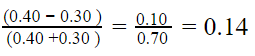The NDVI value of 0.14 indicates that vegetation in this area is very sparse. Since the numbers in the calculation are percentages expressed as decimal numbers, they are already dimensionless. The units used to calculate the percentages already canceled out. Therefore, NDVI has no unit. Rather, it is an index value in which higher values (0.4 to 0.9) show lands covered by green, leafy vegetation and lower values (0 to 0.4) show lands where there is little or no vegetation. Negative values tend to indicate the presence of water, clouds or snow. Because there is no unit, it is dimensionless.

Why is NDVI Important?

NDVI helps us monitor vegetation to:

• monitor the health of vegetation
• monitor ecosystems for disturbances
• determine where vegetation is thriving
• identify where plants are under stress

#### Steps

Let's Focus on Preservation not Deforestation | Video Length 3:03 | https://www.youtube.com/watch?v=3eaAZaAn8JA

1. Watch the Let’s Focus on Preservation not Deforestation video. Two minutes into the video, the formula for calculating NDVI is given.
1. Why is NDVI dimensionless?
2. How is NDVI used to help determine changes in the forest?
3. Calculate the following NDVI ratios.
1. Reflected near infrared light 0.5, Reflectance of red-light 0.06
2. Reflected near infrared light 0.4, Reflectance of red-light 0.25
4. Which ratio above shows green, leafier vegetation? Sparser vegetation?

Sources

1. Dunbar, Brian. “NDVI: Satellites Could Help Keep Hungry Populations Fed as Climate Changes.” NASA, NASA, https://www.nasa.gov/topics/earth/features/obscure_data.html.
2. “Let's Focus on Preservation Not Deforestation.” NASA, NASA, https://develop.larc.nasa.gov/2018/summer/HondurasEco.html. Accessed 24 Apr. 2022.

### Teacher Note

Teachers, these mini lessons/student activities are perfect "warm up" tasks that can be used as a hook, bellringer, exit slip, etc. They take less than a class period to complete. Learn more on the "My NASA Data What are Mini Lessons?" page.

Teachers who are interested in receiving the answer key, please complete the Teacher Key Request and Verification Form. We verify that requestors are teachers prior to sending access to the answer keys as we’ve had many students try to pass as teachers to gain access.

• 6-8
• 9-12

• 15 minutes